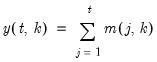Command Reference : Matrix Language Reference

 @colcumsum
Compute cumulative sums for each column of a matrix.
Syntax: @colcumsum(m)
m: matrix, vector
Return: matrix, vector
Returns a matrix where each column contains the cumulative sums of the values of the corresponding column of m.
For each element of the output, compute the cumulative sum of the values in m from the start of the column up to the current row:Examples
matrix m1 = @mnrnd(10, 3)
matrix cumsum = @colcumsum(m1)
computes the cumulative sum for each column of matrix M1 and places the results in CUMSUM.
Cross-references